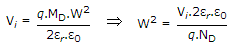# Electronics and Communication Engineering - Electronic Devices and Circuits

6.
An one sided abrupt junction has 1021/m3 of dopants on the lightly doped side, zero bias voltage and a built-in potential of 0.2 V. The depletion width of abrupt junction.(q = 1.6 x 10-19 C, εr =16, ε0 = 8.87 x 10-12 F/m) is
0.036 nm
0.6 μm
3 mm
1.5 mm
Explanation:.

7.
n-type semiconductors
are negatively charged
are produced when indium is added as impurity to germanium
are produced when phosphorus is added as impurity to silicon
none of the above
Explanation:

n type semiconductor is produced when pentavalent impurity is added.

8.
In all metals
conductivity decreases with increase in temperature
current flow by electrons as well as by holes
resistivity decreases with increase in temperature
the gap between valence and conduction bands is small
Explanation:

In all metals conductivity decreases (and resistance increases) with increase in temperature.

9.
The voltage across a zener diode
is constant in forward direction
is constant in reverse direction
is constant in both forward and reverse directions
none of the above
Explanation:

Zener diode is always reverse biased.

10.

Assertion (A): Two transistors one n-p-n and the other p-n-p are identical in all respects (doping, construction, shape, size). The n-p-n transistor will have better frequency response.

Reason (R): The electron mobility is higher than hole mobility.

Both A and R are true and R is correct explanation of A
Both A and R are true but R is not a correct explanation of A
A is true but R is false
A is false but R is true